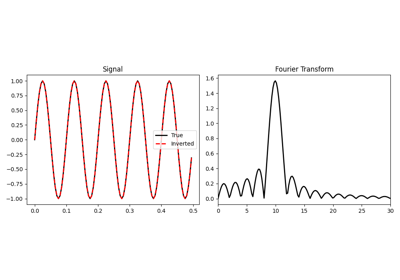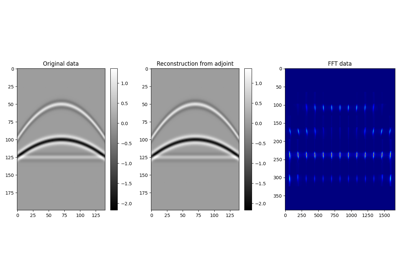# pylops.signalprocessing.FFTND#

pylops.signalprocessing.FFTND(dims, axes=(-3, -2, -1), nffts=None, sampling=1.0, norm='ortho', real=False, ifftshift_before=False, fftshift_after=False, engine='scipy', dtype='complex128', name='F')[source]#

N-dimensional Fast-Fourier Transform.

Apply N-dimensional Fast-Fourier Transform (FFT) to any n axes of a multi-dimensional array.

Using the default NumPy engine, the FFT operator is an overload to either the NumPy numpy.fft.fftn (or numpy.fft.rfftn for real models) in forward mode, and to numpy.fft.ifftn (or numpy.fft.irfftn for real models) in adjoint mode, or their CuPy equivalents. Alternatively, when the SciPy engine is chosen, the overloads are of scipy.fft.fftn (or scipy.fft.rfftn for real models) in forward mode, and to scipy.fft.ifftn (or scipy.fft.irfftn for real models) in adjoint mode.

When using real=True, the result of the forward is also multiplied by $$\sqrt{2}$$ for all frequency bins except zero and Nyquist along the last axes, and the input of the adjoint is multiplied by $$1 / \sqrt{2}$$ for the same frequencies.

For a real valued input signal, it is advised to use the flag real=True as it stores the values of the Fourier transform of the last axis in axes at positive frequencies only as values at negative frequencies are simply their complex conjugates.

Parameters
dimstuple

Number of samples for each dimension

axesint, optional

New in version 2.0.0.

Axes (or axis) along which FFTND is applied

nfftstuple or int, optional

Number of samples in Fourier Transform for each axis in axes. In case only one dimension needs to be specified, use None for the other dimension in the tuple. An axis with None will use dims[axis] as nfft. When supplying a tuple, the length must agree with that of axes. When a single value is passed, it will be used for all axes. As such the default is equivalent to nffts=(None, ..., None).

samplingtuple or float, optional

Sampling steps for each direction. When supplied a single value, it is used for all directions. Unlike nffts, any None will not be converted to the default value.

norm{“ortho”, “none”, “1/n”}, optional

New in version 1.17.0.

• “ortho”: Scales forward and adjoint FFT transforms with $$1/\sqrt{N_F}$$, where $$N_F$$ is the number of samples in the Fourier domain given by product of all elements of nffts.

• “none”: Does not scale the forward or the adjoint FFT transforms.

• “1/n”: Scales both the forward and adjoint FFT transforms by $$1/N_F$$.

Note

For “none” and “1/n”, the operator is not unitary, that is, the adjoint is not the inverse. To invert the operator, simply use Op \ y.

realbool, optional

Model to which fft is applied has real numbers (True) or not (False). Used to enforce that the output of adjoint of a real model is real. Note that the real FFT is applied only to the first dimension to which the FFTND operator is applied (last element of axes)

ifftshift_beforetuple or bool, optional

New in version 1.17.0.

Apply ifftshift (True) or not (False) to model vector (before FFT). Consider using this option when the model vector’s respective axis is symmetric with respect to the zero value sample. This will shift the zero value sample to coincide with the zero index sample. With such an arrangement, FFT will not introduce a sample-dependent phase-shift when compared to the continuous Fourier Transform. When passing a single value, the shift will the same for every direction. Pass a tuple to specify which dimensions are shifted.

fftshift_aftertuple or bool, optional

New in version 1.17.0.

Apply fftshift (True) or not (False) to data vector (after FFT). Consider using this option when you require frequencies to be arranged naturally, from negative to positive. When not applying fftshift after FFT, frequencies are arranged from zero to largest positive, and then from negative Nyquist to the frequency bin before zero. When passing a single value, the shift will the same for every direction. Pass a tuple to specify which dimensions are shifted.

enginestr, optional

New in version 1.17.0.

Engine used for fft computation (numpy or scipy).

dtypestr, optional

Type of elements in input array. Note that the dtype of the operator is the corresponding complex type even when a real type is provided. In addition, note that the NumPy backend does not support returning dtype different than complex128. As such, when using the NumPy backend, arrays will be force-cast to types corresponding to the supplied dtype. The SciPy backend supports all precisions natively. Under both backends, when a real dtype is supplied, a real result will be enforced on the result of the rmatvec and the input of the matvec.

namestr, optional

New in version 2.0.0.

Name of operator (to be used by pylops.utils.describe.describe)

Raises
ValueError
• If nffts or sampling are not either a single value or tuple with the same dimension axes.

• If norm is not one of “ortho”, “none”, or “1/n”.

NotImplementedError

If engine is neither numpy, nor scipy.

FFT

One-dimensional FFT

FFT2D

Two-dimensional FFT

Notes

The FFTND operator (using norm="ortho") applies the N-dimensional forward Fourier transform to a multi-dimensional array. Considering an N-dimensional signal $$d(x_1, \ldots, x_N)$$. The FFTND in forward mode is:

$D(k_1, \ldots, k_N) = \mathscr{F} (d) = \frac{1}{\sqrt{N_F}} \int\limits_{-\infty}^\infty \cdots \int\limits_{-\infty}^\infty d(x_1, \ldots, x_N) e^{-j2\pi k_1 x_1} \cdots e^{-j 2 \pi k_N x_N} \,\mathrm{d}x_1 \cdots \mathrm{d}x_N$

Similarly, the three-dimensional inverse Fourier transform is applied to the Fourier spectrum $$D(k_z, k_y, k_x)$$ in adjoint mode:

$d(x_1, \ldots, x_N) = \mathscr{F}^{-1} (D) = \frac{1}{\sqrt{N_F}} \int\limits_{-\infty}^\infty \cdots \int\limits_{-\infty}^\infty D(k_1, \ldots, k_N) e^{-j2\pi k_1 x_1} \cdots e^{-j 2 \pi k_N x_N} \,\mathrm{d}k_1 \cdots \mathrm{d}k_N$

where $$N_F$$ is the number of samples in the Fourier domain given by the product of the element of nffts. Both operators are effectively discretized and solved by a fast iterative algorithm known as Fast Fourier Transform. Note that the FFTND operator (using norm="ortho") is a special operator in that the adjoint is also the inverse of the forward mode. For other norms, this does not hold (see norm help). However, for any norm, the N-dimensional Fourier transform is Hermitian for real input signals.

Attributes
dimsdtuple

Shape of the array after the forward, but before linearization.

For example, y_reshaped = (Op * x.ravel()).reshape(Op.dimsd).

fstuple

Each element of the tuple corresponds to the Discrete Fourier Transform sample frequencies along the respective direction given by axes.

realbool

When True, uses rfftn/irfftn

rdtypebool

Expected input type to the forward

cdtypebool

Output type of the forward. Complex equivalent to rdtype.

shapetuple

Operator shape

clinearbool

New in version 1.17.0.

Operator is complex-linear. Is false when either real=True or when dtype is not a complex type.

explicitbool

Operator contains a matrix that can be solved explicitly (True) or not (False)

## Examples using pylops.signalprocessing.FFTND`#Fourier Transform

Fourier TransformPatching

Patching